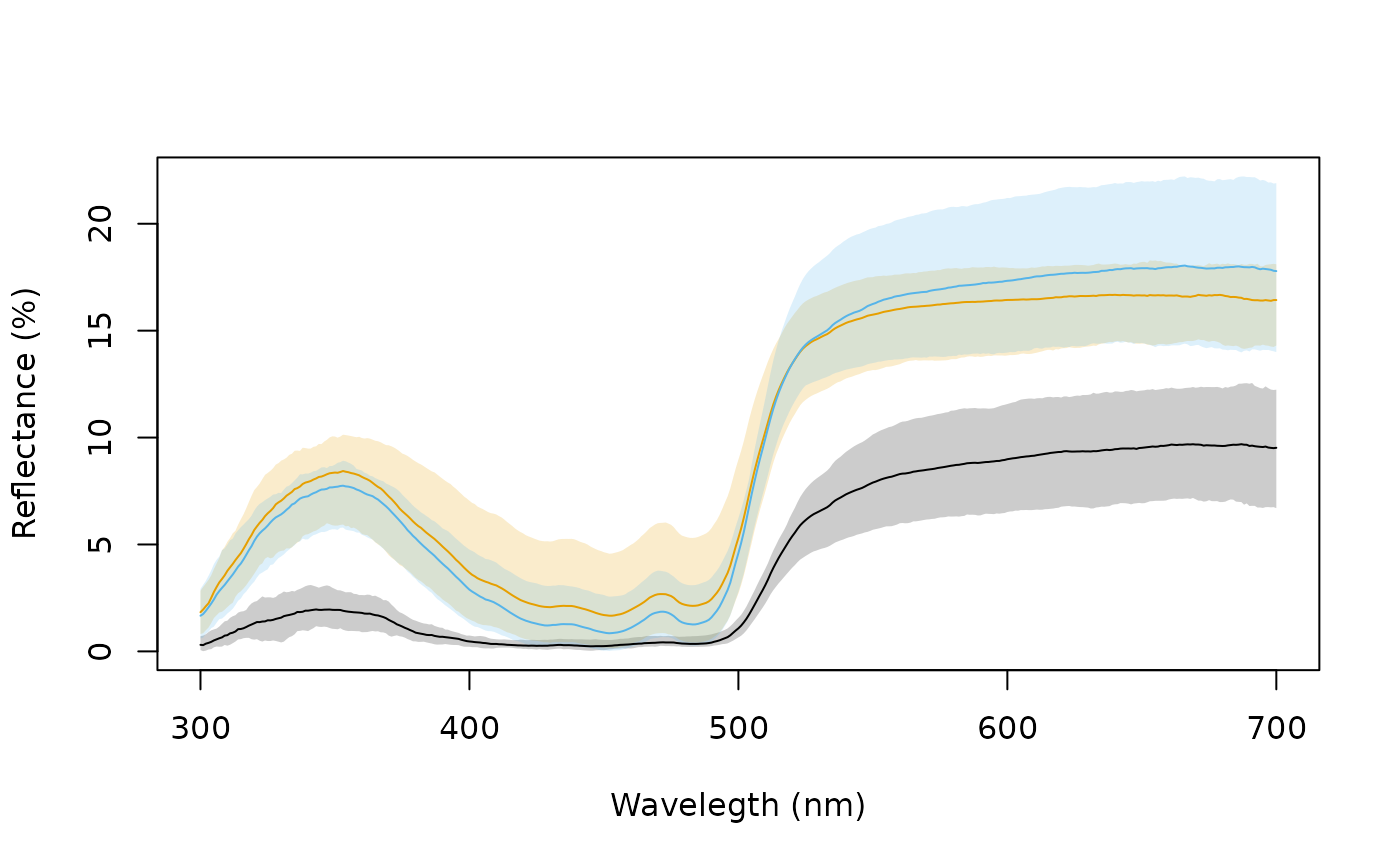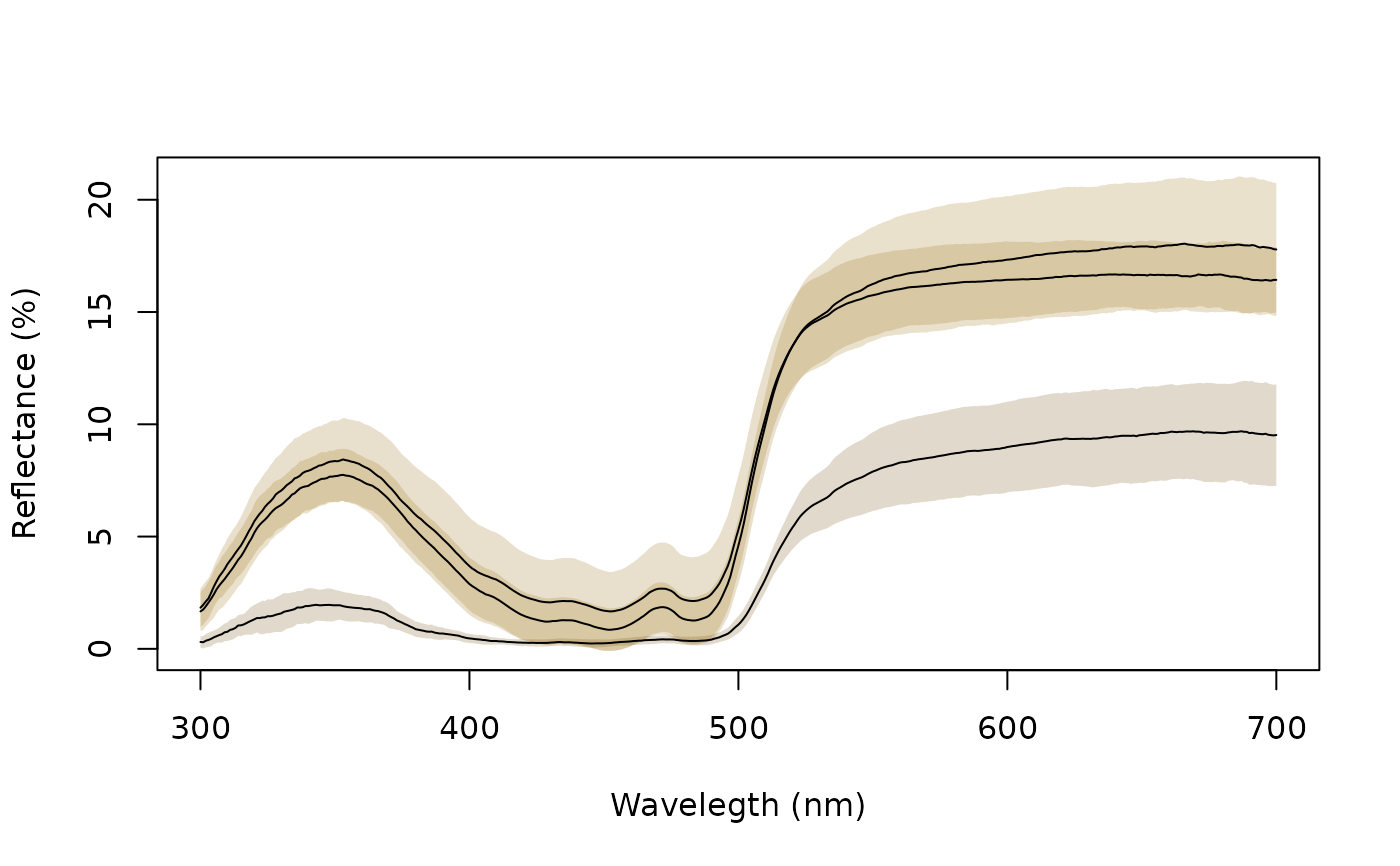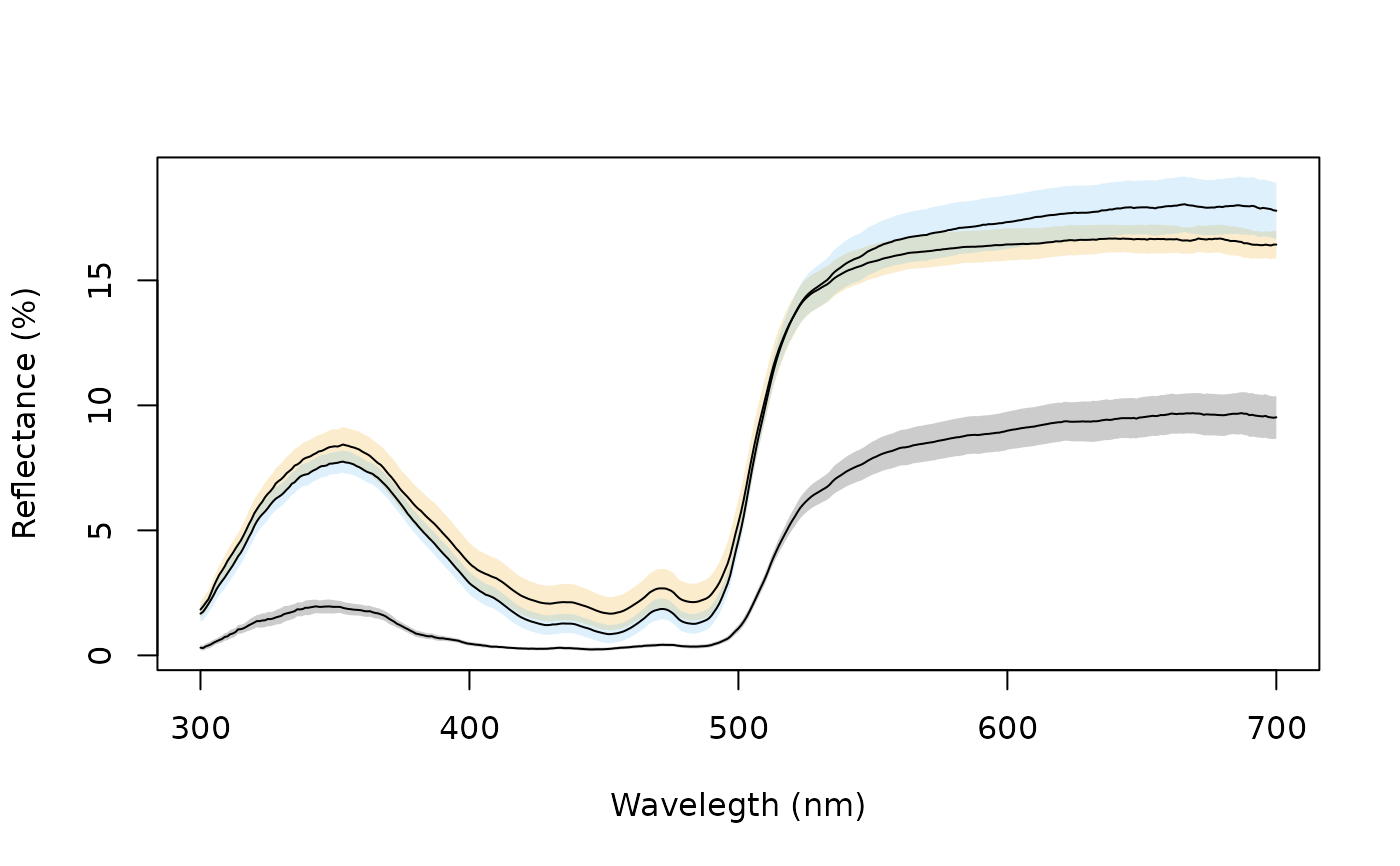Combines and plots spectra (by taking the average and the standard deviation, for example) according to an index or a vector of identities.

aggplot(
rspecdata,
by = NULL,
FUN.center = mean,
FUN.error = sd,
lcol = NULL,
alpha = 0.2,
legend = FALSE,
...
)

## Arguments

rspecdata

(required) a data frame, possibly of class rspec, which contains a column containing a wavelength range, named 'wl', and spectra data in remaining columns.

by

(required) either a single value specifying the range of spectra within the data frame to be combined (for example, by = 3 indicates the function will be applied to groups of 3 consecutive columns in the spectra data frame); a vector containing identifications for the columns in the spectra data frame (in which case the function will be applied to each group of spectra sharing the same identification); or a list of vectors, e.g., by = list(sex, species).

FUN.center

the function to be applied to the groups of spectra, calculating a measure of central tendency (defaults to base::mean()).

FUN.error

the function to be applied to the groups of spectra, calculating a measure of variation (defaults to stats::sd()).

lcol

colour of plotted lines indicating central tendency.

colour of shaded areas indicating variance measure.

alpha

legend

...

additional graphical parameters to be passed to plot.

## Value

Plot containing the lines and shaded areas of the groups of spectra.

## Author

Rafael Maia rm72@zips.uakron.edu

## Examples


data(sicalis)

# Create grouping variable based on spec names
bysic <- gsub("^ind[0-9].", "", names(sicalis)[-1])

# Plot using various error functions and options
aggplot(sicalis, bysic)aggplot(sicalis, bysic, FUN.error = function(x) quantile(x, c(0.0275, 0.975)))aggplot(sicalis, bysic, shadecol = spec2rgb(sicalis), lcol = 1)aggplot(sicalis, bysic, lcol = 1, FUN.error = function(x) sd(x) / sqrt(length(x)))